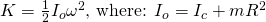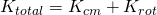# Physics - Classical Mechanics - Rotation about a moving axis (Rolling motion)[Image 1]

## Introduction

Hey it's a me again @drifter1! Today we continue with Physics and more specifically the branch "Classical Mechanics" to get into the Rotation about a moving axis, which can also be called Rolling motion. So, without further ado, let's get straight into it!

## Fixed Axis Rotation

Until now we mostly talked about Translational and Angular motion separately. So, an object either moved from one point to another in space (translational motion) or rotated about it's axis that was also a fixed point (angular motion). The first part has been covered a lot in earlier articles of the series. The second part is what we are covering right now in the series. Combining the terms and quantities that we studied in the previous articles about Angular motion, right now we know that an object rotates:
• around a fixed axis of rotation 'O', called the pivot point
• because of a change in it's angular velocity (ω) that has been caused by a Torque (τ) that made it accelerate with some angular acceleration (a). Also think about: Στ = Ia
• wanting to keep it's "rotation state" (rotating or not)
If an object simply rotates around a fixed axis then we can define it's total kinetic energy to be equal to it's angular kinetic energy. Let's consider a rigid body with some mass m and radius R and that we want to calculate the kinetic energy at the point of contact to the ground (will help us transition to rolling motion). Of course, we have to apply the Parallel Axis Theorem to get the Moment of Inertia about the point of contact. So, the angular kinetic energy is given by:## Moving Axis Rotation

Let's now think about the combined motion of translation + rotation (rolling motion). Continuing with the expansion of the previous equation we get:So, you can see the that kinetic energy at the point of contact is equal to the sum of Angular/Rotational and Translational kinetic energy. In this previous equation the translational motion part is of course equal to the kinetic energy of the center-of-mass (CM) and so we can also write that the total kinetic energy of a rolling body is:This means that calculating the kinetic energy of a rolling body is quite simple! We just have to calculate the kinetic energy of it's center-of-mass and add it to the angular kinetic energy in respect to the center-of-mass. This means that we only have to know the moment of Inertia (I) about the center-of-mass, which can be found easily for common shapes.

Of course, the previous equation can be quite misleading and that's why I made the complete proof using the moment of inertia around the point of contact, where we also had to apply the Parallel Axis Theorem. We never really added the impact of the translational motion, we just tried to calculate the kinetic energy at some point different than the center-of-mass (and mostly axis of rotation). Talking about a distance of R the substitution of the term ωR gave us the tangential velocity v (which is also equal to the velocity of the center-of-mass) and so, in the end, the kinetic energy of the center-of-mass (Kcm = ½mv2). Stay Cautious!

## Final words | Next up

This is actually it for today's post! Next time we will continue with the definition of Work and Power in Angular Motion....

See ya!Keep on drifting!

H2
H3
H4
3 columns
2 columns
1 column